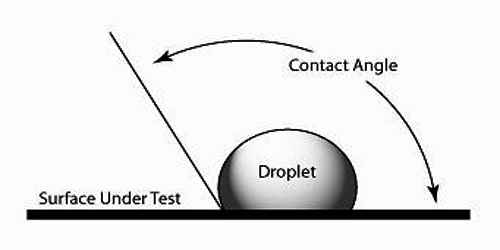# Angle of Contact

Angle of Contact

Definition: The angle which the tangent to the liquid surface at the point of contact makes with the solid surface of the liquid is called the angle of contact.

When a liquid comes in contact with a solid surface it is generally curved at the point of contact. There exists an angle between these two contact surfaces. Apparently, this angle is the contact angle. Now we will explain actually what is the angle of contact.

Explanation: When a metal plate is dipped vertically in water or in any liquid, it is found that water or liquid in immediate contact with the metal plate becomes slightly curved. In addition to the cohesive force between molecules of the liquid, there exists an adhesive force between the solid surface and the liquid. In this case, the mutual attractive force between the molecules of the same material is the cohesive force. This force obeys the inverse square law of distance.On the other hand, the mutual attractive force between the different molecules is the adhesive force. The cohesive force tries to keep the liquid. surface horizontal. On the other hand, the adhesive force tries to raise the liquid surface. Due to the action of the resultant of these two forces the liquid either rise [Fig (a)] along the surface of the solid or gets depressed downward [Fig (b)]. If a tangent is drawn at the point of contact, it makes two angles with the surface of solid. The angle made by the tangent with the surface of the solid inside the liquid is called angle of contact.